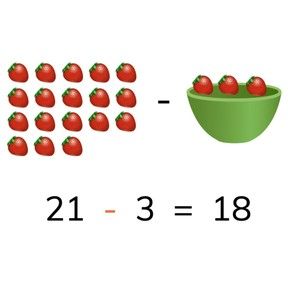Subtraction to 30 with regrouping

# Subtraction to 30 with regrouping8,000 schools use Gynzy92,000 teachers use Gynzy1,600,000 students use Gynzy

## General

Students learn to subtract numbers to 30 regrouping tens.

2.OA.A.1

## Relevance

It is important to be able to subtract numbers to 30, to be able to determine how many you have left.

## Introduction

Start by asking students to determine which ball has the number shown by the question mark on the number line on the interactive whiteboard. Then practice a subtraction problem to 20 with students on the number line.

## Development

Start by discussing the importance of being able to subtract to 30 and explain how to calculate to the ten. The learning goal is then presented in three ways, visually, in the abstract, and in story form. You can select the method which best applies to your classroom situation by using the blue menu in the bottom right of the page. Otherwise, you can start at the first page with the visual support. Use the image of fruit to explain how to subtract the two numbers. Then have students practice the next set on their own. Then the abstract subtraction problem is given. Tell students that they can solve these by counting back in their heads, or by imagining a number line, or even by using blocks or a rekenrek. Discuss with students that it is useful to first count back to the ten, and then to take away the rest. Have students practice with a set of abstract subtraction problems. Then the steps of solving a story problem are given, discuss these step by step and solve the given story problem together. Then ask students to work in pairs and determine the difference in the next story problem. Discuss their answers as a class. Each method has the teacher explain first, then practice together, and then has the students practice individually to check their understanding.

Check that students are able to subtract to 30 regrouping tens by asking the following questions:
- Why is it useful to be able to subtract to 30?
- How do you determine which number is first in a subtraction problem?
- How do you regroup tens?

## Guided practice

Students are given an abstract problem without visual support, and are then given story problems to solve. Ask how they solved the problems.

## Closing

Do one of each kind of subtraction problems with the students (visual, abstract, and story). Then divide the class into groups. Give each group three dice. At the start of the first round each student starts with 30 points. Each student throws the three dice and subtracts those numbers from their total of 30 points. The round ends when each student in the group has subtracted their numbers from 30. The student who has the most points left, is the winner of the round. The second round, students start with 29 points, and in the third round, they start with 28 points (etc).

## Teaching tips

Students who have difficulty subtracting numbers to 30 can be supported by the use of manipulatives, like blocks. To help students regroup the tens, have students draw the subtraction problem on the number line, and then have them count back to the previous ten, and then count on.

## Instruction materials

3 dice per group
Optional: blocks or other manipulatives

### The online teaching platform for interactive whiteboards and displays in schools

• Save time building lessons

• Manage the classroom more efficiently

• Increase student engagement